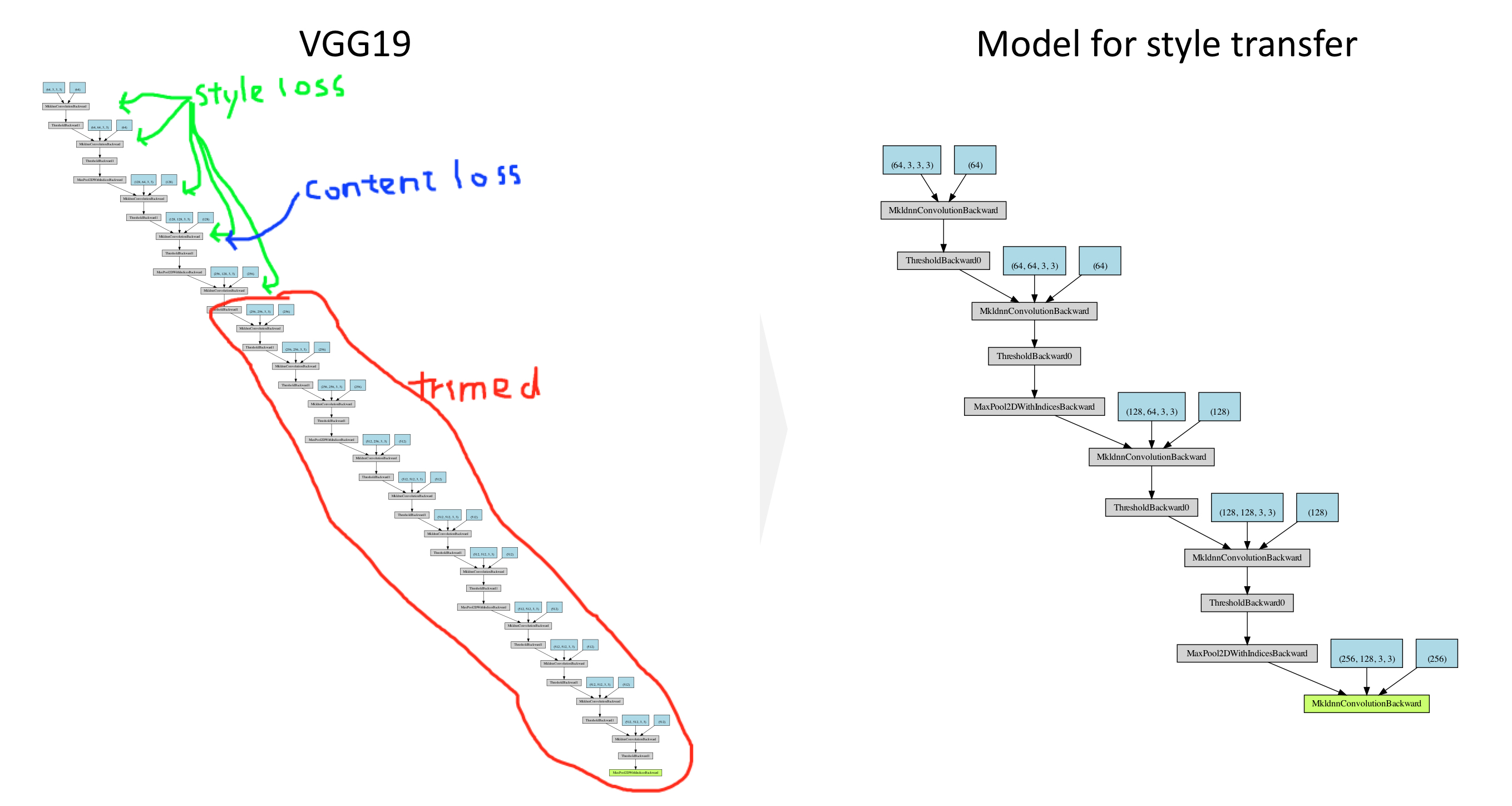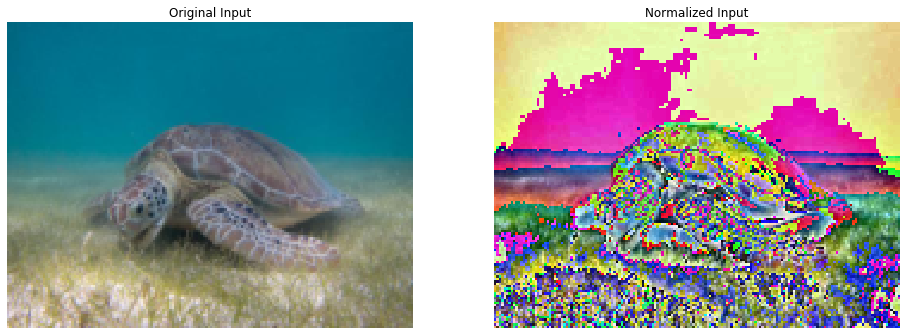# Style Transfer using Pytorch (Part 3)

## Goal¶

This post aims to explain the concept of style transfer step-by-step. Part 3 is about building a modeling for style transfer from VGG19.Reference

## Libraries¶

In :
import pandas as pd
import copy

# Torch & Tensorflow
import torch
import torch.nn as nn
import torch.nn.functional as F
import torchvision
import tensorflow as tf

# Visualization
from torchviz import make_dot
from PIL import Image
import matplotlib.pyplot as plt
%matplotlib inline


## Configuration¶

In :
device = torch.device("cuda" if torch.cuda.is_available() else "cpu")


## Functions¶

The functions covered by the previous posts (Part 1, Part 2) are as follows.

### Functions from Part 1¶

In :
# desired size of the output image
imsize = (512, 512) if torch.cuda.is_available() else (128, 128)  # use small size if no gpu

torchvision.transforms.Resize(imsize),  # scale imported image
torchvision.transforms.ToTensor()])  # transform it into a torch tensor

image = Image.open(image_name)

# fake batch dimension required to fit network's input dimensions
return image.to(device, torch.float)

In :
unloader = torchvision.transforms.ToPILImage()

def imshow_tensor(tensor, ax=None):
image = tensor.cpu().clone()  # we clone the tensor to not do changes on it
image = image.squeeze(0)      # remove the fake batch dimension

if ax:
ax.imshow(image)
else:
plt.imshow(image)


### Functions from Part 2¶

In :
class ContentLoss(nn.Module):

def __init__(self, target,):
super(ContentLoss, self).__init__()
self.target = target.detach()

def forward(self, input):
self.loss = F.mse_loss(input, self.target)
return input

def gram_matrix(input):
# Get the size of tensor
# a: batch size
# b: number of feature maps
# c, d: the dimension of a feature map
a, b, c, d = input.size()

# Reshape the feature
features = input.view(a * b, c * d)

# Multiplication
G = torch.mm(features, features.t())

# Normalize
G_norm = G.div(a * b * c * d)
return G_norm

class StyleLoss(nn.Module):

def __init__(self, target_feature):
super(StyleLoss, self).__init__()
self.target = gram_matrix(target_feature).detach()

def forward(self, input):
G = gram_matrix(input)
self.loss = F.mse_loss(G, self.target)
return input


In :
d_path = {}


## Modeling¶

### Load a pre-trained CNN model - VGG19¶

In :
cnn = torchvision.models.vgg19(pretrained=True).features.to(device).eval()


### Visualize the model¶

In :
x = torch.zeros(1, 3, 224, 224, dtype=torch.float, requires_grad=False)
out = cnn(x)
make_dot(out)

Out:

### Modify the pre-trained model for style transfer¶

#### Add normalization for input images¶

This is specific normalization required for VGG networks. Each channel for input images should be normalized by each channel normalized by mean=[0.485, 0.456, 0.406] and std=[0.229, 0.224, 0.225]

In :
cnn_normalization_mean = torch.tensor([0.485, 0.456, 0.406]).to(device)
cnn_normalization_std = torch.tensor([0.229, 0.224, 0.225]).to(device)

# create a module to normalize input image so we can easily put it in a
# nn.Sequential
class Normalization(nn.Module):
def __init__(self, mean, std):
super(Normalization, self).__init__()
# .view the mean and std to make them [C x 1 x 1] so that they can
# directly work with image Tensor of shape [B x C x H x W].
# B is batch size. C is number of channels. H is height and W is width.
self.mean = torch.tensor(mean).view(-1, 1, 1)
self.std = torch.tensor(std).view(-1, 1, 1)

def forward(self, img):
# normalize img
return (img - self.mean) / self.std


#### Example of normalization¶

We use the functions image_loader and imshow, which is named here as imshow_tensor for tensor covered by Style Transfer using Pytorch (Part 1) to get the image as tensor.

In :
import warnings
warnings.filterwarnings("ignore")

# Normalize
normalize = Normalization(cnn_normalization_mean, cnn_normalization_std).to(device)
img_norm = normalize.forward(img)

# Visualize
fig, axes = plt.subplots(1, 2, figsize=(16, 8))
imshow_tensor(img, ax=axes);
axes.set_title('Original Input'); axes.axis('off');
imshow_tensor(img_norm, ax=axes);
axes.set_title('Normalized Input'); axes.axis('off');### Create a sequential model for style transfer¶

In :
# desired depth layers to compute style/content losses :
content_layers_default = ['conv_4']
style_layers_default = ['conv_1', 'conv_2', 'conv_3', 'conv_4', 'conv_5']

def get_style_model_and_losses(cnn, normalization_mean, normalization_std,
style_img, content_img,
content_layers=content_layers_default,
style_layers=style_layers_default):
cnn = copy.deepcopy(cnn)

# normalization module
normalization = Normalization(normalization_mean, normalization_std).to(device)

# just in order to have an iterable access to or list of content/syle
# losses
content_losses = []
style_losses = []

# assuming that cnn is a nn.Sequential, so we make a new nn.Sequential
# to put in modules that are supposed to be activated sequentially
model = nn.Sequential(normalization)

i = 0  # increment every time we see a conv
for n_child, layer in enumerate(cnn.children()):
print()
print(f"n_child: {n_child}")
if isinstance(layer, nn.Conv2d):
i += 1
name = 'conv_{}'.format(i)
elif isinstance(layer, nn.ReLU):
name = 'relu_{}'.format(i)
# The in-place version doesn't play very nicely with the ContentLoss
# and StyleLoss we insert below. So we replace with out-of-place
# ones here.
layer = nn.ReLU(inplace=False)
elif isinstance(layer, nn.MaxPool2d):
name = 'pool_{}'.format(i)
elif isinstance(layer, nn.BatchNorm2d):
name = 'bn_{}'.format(i)
else:
raise RuntimeError('Unrecognized layer: {}'.format(layer.__class__.__name__))

print(f'Name: {name}')
if name in content_layers:
target = model(content_img).detach()
content_loss = ContentLoss(target)
content_losses.append(content_loss)

if name in style_layers:
target_feature = model(style_img).detach()
style_loss = StyleLoss(target_feature)
style_losses.append(style_loss)

# now we trim off the layers after the last content and style losses
for i in range(len(model) - 1, -1, -1):
if isinstance(model[i], ContentLoss) or isinstance(model[i], StyleLoss):
break

model = model[:(i + 1)]

return model, style_losses, content_losses

In :
style_img = image_loader(d_path['content'])
assert style_img.size() == content_img.size(), \
"we need to import style and content images of the same size"

In :
# Obtain the model for style transfer
model, style_losses, content_losses = get_style_model_and_losses(cnn, cnn_normalization_mean, cnn_normalization_std, style_img, content_img)

n_child: 0
Name: conv_1

n_child: 1
Name: relu_1

n_child: 2
Name: conv_2

n_child: 3
Name: relu_2

n_child: 4
Name: pool_2

n_child: 5
Name: conv_3

n_child: 6
Name: relu_3

n_child: 7
Name: conv_4

n_child: 8
Name: relu_4

n_child: 9
Name: pool_4

n_child: 10
Name: conv_5

n_child: 11
Name: relu_5

n_child: 12
Name: conv_6

n_child: 13
Name: relu_6

n_child: 14
Name: conv_7

n_child: 15
Name: relu_7

n_child: 16
Name: conv_8

n_child: 17
Name: relu_8

n_child: 18
Name: pool_8

n_child: 19
Name: conv_9

n_child: 20
Name: relu_9

n_child: 21
Name: conv_10

n_child: 22
Name: relu_10

n_child: 23
Name: conv_11

n_child: 24
Name: relu_11

n_child: 25
Name: conv_12

n_child: 26
Name: relu_12

n_child: 27
Name: pool_12

n_child: 28
Name: conv_13

n_child: 29
Name: relu_13

n_child: 30
Name: conv_14

n_child: 31
Name: relu_14

n_child: 32
Name: conv_15

n_child: 33
Name: relu_15

n_child: 34
Name: conv_16

n_child: 35
Name: relu_16

n_child: 36
Name: pool_16

In :
x = torch.zeros(1, 3, 128, 128, dtype=torch.float, requires_grad=False)
make_dot(model(x))

Out: